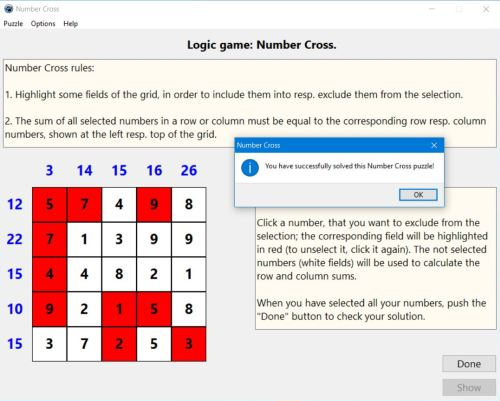# Computing: Free Pascal Programming

 Programming Home Home Contact

## Logic puzzle: Number Cross (NumberCross).

Description: Simple puzzle game with numbers placed on a grid, the aim being to find those numbers, that added by row and column, equal a given row/column sum. Even though the application may be considered to be a mathematical game (with basic arithmetic calculation), it primarily is a logic puzzle. In fact, to find the correct solution of the puzzle, you have to explore the grid numbers, and by logical thinking, determine, those numbers, that are mandatorily part of the ones to be used to get the row/column sums or, on the contrary, those, that can't be part of this selection.
The puzzle is normally done by marking the numbers to be excluded, i.e. that will not be used to get the row/column sums. NumberCross gives the choice ("Options" menu), to select the numbers to be excluded, or those to be included.

Free Pascal features: Changing the color of shapes and the caption of static-texts during execution. Two-dimensional arrays (classic Pascal).

Screenshot: